background

# Lattice Points of Lines

Lecture 2

In lecture one, we introduced the concept of counting the number of lattice points that lie inside and on the boundary of a given circle of radius. We also mentioned that, counting the number of lattice points in curvy regions such as hyperbolas, is equivalent to determining whether a given integeris prime or not. Since, it is difficult to check the primality of a given number, this implies that counting the number of lattice points that lie within and on the boundary of curvy regions, is as difficult as checking the primality of an integer number. As a result, we can safely deduce that counting the number of lattice points inside and on the boundary of curvy regions is a fairly complicated process. Suppose then we restrict ourselves to counting the number of lattice points of less curvy regions such as lines for instance.

Lattice Points of lines

Suppose we have a line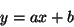Again a natural question arises, how many lattice points are on it? To study this question further we offer the following theorem:

theorem 1   Every line is of the following types:
1. Rational slope no lattice points
2. Rational slope infinitely many lattice points
3. Irrational slope no lattice points
4. Irrational slope exactly one lattice point
5. Lines parallel to theoraxis infinitely many lattice points depending on whether the constant k in,is integral or not.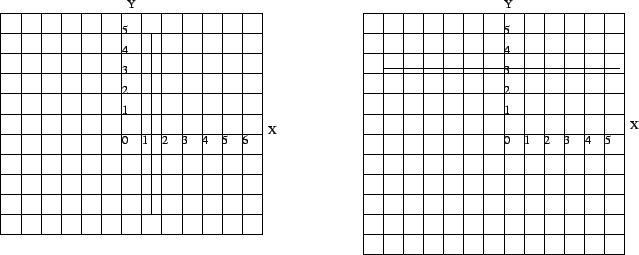If we look at figure one we will see that the line skips every integral point on the graph. Suppose somebody said that the line should cross through (1,1). Then we can easily disprove such claim by plainly substitutingfor 1 and solving for. So,. A non-integral solution. In this manner we can show that such line cannot have any lattice points. In fact given any line of the form,, s.t. the line has a rational slope and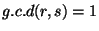, wheredoes not divide. We will get that such a line will not have any lattice points on it. However, if we make the slope rational then we will more thatn likely have a lattice point as figure 3 shows. However, as we have seen from figure 2, having a rational slope does not guarantee that a line have any lattice points, to guarantee at least one lattice point consider the following theorem

theorem 2   Ifhas a rational slope and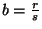s.t.andthenat least one lattice point on the line

Now if we look at figure 4 we claim that such graph does not have any lattice points on it. However, the point (1,4) looks like an integral solution. However this cannot be for,an integral solution. Now in figure 5 we claim that (0,0) is the only lattice point on that graph, if we look closely (-1,-1) looks like a lattice point on the graph, but as previously done,. We can also say that the point (4,5) is also a point on the graph of figure 5. But the following calculation shows that this cannot be,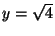,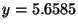a non-integral solution.

Suppose now we wanted to show that a line contains no lattice points! the following theorem can help with this

theorem 3   Ifwhere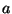is irrational,is a rational non-integer, then the line has no lattice points

Moreover, given a line, whereis irrational andis any real number, has on either side an infinite strip of lattice points lying close to the respective line.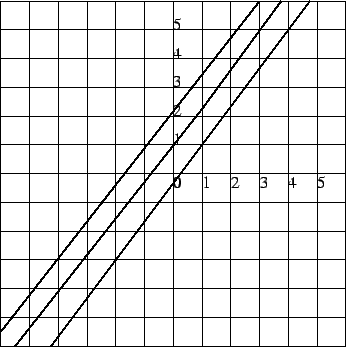If we consider figure 7 you will notice that on either side there exists lattice points trapped inside the infinite strip. For instance, the lattice points (1,2), (2,3) are on the right side of the line and the points (1,3) and (2,4) exist on the left side of the line. In this manner we can find lattice points inside the parallel strip for the entire line.

• Mathematics • The University of California, Davis •
• One Shields Avenue • Davis, CA 95616 •